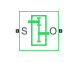# Gear Box

Gear box in mechanical systems

•Libraries:
Simscape / Foundation Library / Mechanical / Mechanisms

## Description

The Gear Box block represents an ideal, nonplanetary, fixed gear ratio gear box. The gear ratio is determined as the ratio of the input shaft angular velocity to that of the output shaft.

The gear box is described with the following equations:

`${\omega }_{S}=N·{\omega }_{O}$`
`${T}_{O}=N\cdot {T}_{S}$`
`${P}_{S}={\omega }_{S}\cdot {T}_{S}$`
`${P}_{O}=-{\omega }_{O}\cdot {T}_{O}$`

where

• ωS is input shaft angular velocity.

• ωO is output shaft angular velocity.

• N is gear ratio.

• TS is torque on the input shaft.

• TO is torque on the output shaft.

• PS is power on the input shaft.

• PO is power on the output shaft. Notice the minus sign in computing PO. One of the network rules is that the power flowing through a conserving port is positive if it is removed (dissipated) from the circuit, and is negative if the component generates power into the system.

Connections S and O are mechanical rotational conserving ports associated with the box input and output shaft, respectively. The block positive directions are from S to the reference point and from the reference point to O.

### Variables

To set the priority and initial target values for the block variables prior to simulation, use the Initial Targets section in the block dialog box or Property Inspector. For more information, see Set Priority and Initial Target for Block Variables.

Nominal values provide a way to specify the expected magnitude of a variable in a model. Using system scaling based on nominal values increases the simulation robustness. Nominal values can come from different sources, one of which is the Nominal Values section in the block dialog box or Property Inspector. For more information, see Modify Nominal Values for a Block Variable.

## Ports

### Conserving

expand all

Mechanical rotational conserving port associated with the input shaft.

Mechanical rotational conserving port associated with the output shaft.

## Parameters

expand all

The ratio of the input shaft angular velocity to that of the output shaft. You can specify both positive and negative values.

## Version History

Introduced in R2007a